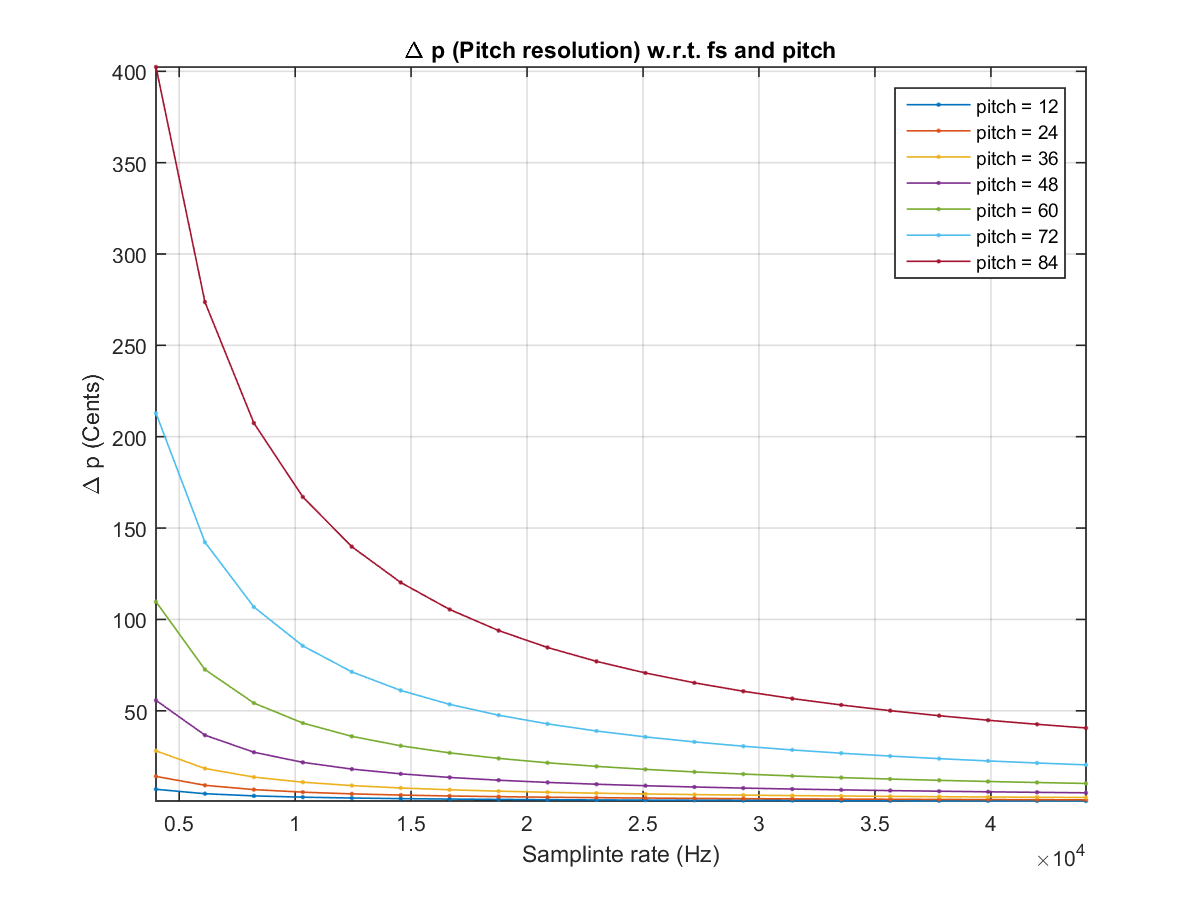## 7-9 How to Increase Pitch Resolution (嚙踝蕭嚙踝蕭嚙踝蕭嚙踝蕭嚙踝蕭嚙踝蕭嚙踝蕭嚙踝蕭嚙踝蕭嚙踝蕭嚙踝蕭嚙踝蕭)

p = 69 + 12*log2(f/440) = 69 + 12*log2((fs/L)/440)

DP = (69 + 12*log2((fs/L)/440)) - (69 + 12*log2((fs/(L+1))/440)) = -12*log2(1+1/L) = -12*log2(1+f/fs)

Example 1: pitchResolution01.m% Pitch resolution w.r.t. sampling rate and pitch fs=linspace(4000, 44100, 20)'; pitch=12*(1:7); deltaP=[]; for i=1:length(pitch) f=440*2^((pitch(i)-69)/12); deltaP=[deltaP, 12*log2(1+f./fs)]; end plot(fs, 100*deltaP, '.-'); axis tight; grid on xlabel('Samplinte rate (Hz)'); ylabel('\Delta p (Cents)'); title('\Delta p (Pitch resolution) w.r.t. fs and pitch'); % Display legends pitchStr={}; for i=1:length(pitch) pitchStr={pitchStr{:}, ['pitch = ', int2str(pitch(i))]}; end legend(pitchStr);Audio Signal Processing and Recognition (音訊處理與辨識)